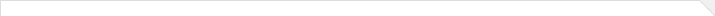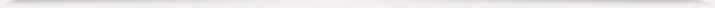报告题目： Dynamic mode decomposition of nonequilibrium Green's function for quantum many-body systems 报 告 人： 印佳 报告人所在单位： Applied Mathematics and Computational Research Division (AMCRD), Lawrance Berkeley National Laboratory 报告日期： 2023-01-06 报告时间： 14:10—14:50 报告地点： Tencent meeting: 922-819-862 报告摘要： Simulating quantum many-body systems away from equilibrium is computationally challenging. To make it easier, a practical way is to examine the Green's function based on the many-body perturbation theory. However, the Kadanoff-Baym equations (KBEs) which describe the dynamics of the two-time non-equilibrium Green's function (NEGF) form a set of coupled nonlinear integro-differential equations difficult to solve. In fact, to propagate the system until time T, typical numerical methods will take O(T^3) computational time. To deal with this problem, I applied DMD, which is a data-driven model order reduction technique, to simulate the long-time dynamics of the NEGF by using snapshots computed within a small time window. This technique was first applied to the time-diagonal of the two-time Green's function, and then to the off-diagonal elements by decomposing the Green's function into a number of one-time functions. The effectiveness of DMD is demonstrated on a two-band Hubbard model system. In the equilibrium limit, the DMD analysis yields results that are consistent with those produced from a linear response analysis. In the nonequilibrium case, the extrapolated dynamics produced by DMD is more accurate than a special Fourier extrapolation scheme. A potential pitfall of the standard DMD method comes from the insufficient spatial/momentum resolution of the discretization scheme. For the model system, this problem can be overcome by using a variant of DMD known as the higher order DMD (HODMD).学术海报6.pdf 本年度学院报告总序号： 716